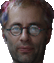<% option explicit dim Rows, Columns, Cells, Number(), n, RandomPic, rs, Row, Column, ColumnWidth, Picture() Rows = 6 Columns = 10 Cells = Rows * Columns ColumnWidth = int (100 / Columns) '''''''''''''''''''''''''''''''''''''''''''''''''''''''''''''''''''''''''''''''''''''''''''''''' sub RefreshNumber dim m, NewNumber(), NewNumberSize redim NewNumber (0) for m = 1 to ubound(number) if not number(m) = 0 then NewNumberSize = ubound(newnumber) + 1 redim preserve NewNumber(NewNumberSize) NewNumber(NewNumberSize) = number (m) end if next 'Number() = Cbyte(NewNumber()) redim Number (ubound(newnumber)) for m = 1 to ubound(newnumber) Number (m) = NewNumber (m) next end sub '''''''''''''''''''''''''''''''''''''''''''''''''''''''''''''''''''''''''''''''''''''''''''''''' redim Number(Cells) for n = 1 to Cells Number (n) = n next reDim Picture(Cells) randomize for n = 1 to Cells RandomPic = int (rnd * ubound(number) + 1) Picture (n) = number(randompic) number (randompic) = 0 RefreshNumber next %> Reality

Reality
by A. Molotkov

<% for Row = 1 to Rows %> <% for Column = 1 to Columns %> <% next %> <% next %>

Click on an image to expand.  Refresh the page to reorder images.

Advanced viewing options (IE 4+ only)